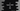# C logical AND, && explanation with examples## C logical AND:

Logical AND or && is a commonly used logical operation like logical OR or logical NOT. AND is used to check if two conditions are true or not. If both are true, then it returns true, else it returns false.

In C programming language, double ampersand character && is used to denote logical AND. It is a binary operator and it needs two operands. Based on these two operands, it returns one result.

Below is the definition:

``case_1 && case_2``

And, below is the truth table for AND :

case_1 case_2 result
true true true
true false false
false true false
false false false

So, as you can see, it results true only if both are true. Other than that, it is false always.

true is 1 and false is 0 in C.

Let’s move to the examples:

### Example 1: Take two numbers from user and check if both are positive or not:

In this problem, we will take two numbers as input from the user and using AND, we will check if both are positive or not. For that, we can use && and check if both numbers are positive or not.

``````#include <stdio.h>

int main()
{
int first, second;

printf("Enter the first number : ");
scanf("%d", &first);

printf("Enter the second number : ");
scanf("%d", &second);

if (first > 0 && second > 0)
{
printf("Both numbers are positive.\n");
}
else
{
printf("Both numbers are not positive\n");
}
}``````

In this example, we have defined two integer variables first and second and read two numbers as user inputs and stored them in these variables.

Using the if condition, we are checking if both of these variables are greater than 0 or not and based on that, we are printing the message that both are positive or not positive.

Sample output of this program:

``````Enter the first number : 10
Enter the second number : 20
Both numbers are positive.

Enter the first number : 10
Enter the second number : -9
Both numbers are not positive``````

As you can see, it runs the printf if both numbers are positive, else it moves to the else block.

### C program to check if a number is divisible by 2, 4 and 8:

This program will show you how we can check if a number is divisible by 2, 4 and 8. Below is the complete program :

``````#include <stdio.h>

int main()
{
int num;

printf("Enter a number : ");
scanf("%d", &num);

if (num % 2 == 0 && num % 4 == 0 && num % 8 == 0)
{
printf("%d is divisible by 2, 4 and 8\n", num);
}
else
{
printf("%d is not divisible by 2, 4 and 8 all\n", num);
}
}``````

Here, we are using one if block with three different cases meets that condition and we are binding them using &&. It will move inside the if block only if all of these are true. Else, it will move to else block.

``````Enter a number : 8
8 is divisible by 2, 4 and 8

Enter a number : 33
33 is not divisible by 2, 4 and 8 all``````